# Problems for 6th grade (the sixth graders) - page 38

1. BenchesThe park has 64 benches. Occupied are by 18 more than empty. How many benches are occupied and empty ?
2. ClassroomOne-eighth of 9th class was interested in studying at a grammar school, at a business academy one sixth, at secondary vocational schools quarter, to SOU one third and the remaining three students were interested in the school of art direction. How many st
3. Cube surface areaWall of cube has content area 99 cm square. What is the surface of the cube?
4. Pedestrian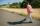Dana started at 10:00 from the point A to point B. These points are distant 12 km. Determine how fast Daniel went when the place B arrived at 11:54. Speed express in km/h.
5. Unknown number 16My number's tens is three times more then ones My number's ones is twice the number of thousands and my number's hundreds is half the number of of tens. I have two ones. which number am I?
6. Excavator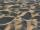The excavator bucket capacity is 0.5 m3. Determine the mass of sand that the excavator picks up. The sand density is 1650 kg/m3.
7. ChocolateChildren break chocolate first to third and then every part of another half. What kind got each child? Draw a picture. What part would have received if each piece have halved?
8. Perimeter to areaCalculate the area of a circle with the perimeter 15 meters.
9. Forest nurseryIn the forest nursery after winter, they found that 1/10 stems died out of them. For them, they land 193 new spruces. How many spruces are in the forest nursery?
10. Square glassWhat is the area of the square window glass with the side 4 dm? Do you know how many cm2 is it?
11. Unknown number 6Determine x if 1/6 of x is equal to 2/5 of the number 24.
12. DivisionWhich number in division 16 give 12 and the rest 3?
13. Cuboid box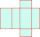Cuboid box have dimensions of 30 cm, 25 cm and 40 cm. Sketch its network and compute surface area of box.
14. NormThree workers planted 3555 seedlings of tomatoes in one dey. First worked at the standard norm, the second planted 120 seedlings more and the third 135 seedlings more than the first worker. How many seedlings were standard norm?
15. Can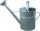Watering can full of water weighs 10 kg. Half-full can weighs 5.5 kg. How much weigh can?
16. Unknown number 11That number increased by three equals three times itself?
17. Waste paper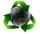Our school attend 1,300 pupils. Each pupil collected plastic bottles or paper. Plastic bottles have collected 930 pupils and 600 pupils old paper. How many students have collected together plastic bottles and waste paper?
18. The bunsKate, Zofia and Peter Liked buns. Even today, their grandmother prepare their favorite meal. Katka eats 4 bunches, Žofia 3 and Petra eats 5 buns. Their grandmother said to them, "My inmate will you know how many buns I have been make today, if those you ea
19. Guess a fractionTom was asked to guess a fraction. The sum of 1/2 the numerator and 1/3 of its denominator is 30. If Tom subtracts 36 from its denominator, the fraction becomes 1/3. What is the fraction that Tom was asked to guess? (Leave your answer in simplest form)
20. LCD 2The least common denominator of 2/5, 1/2, and 3/4

Do you have an interesting mathematical problem that you can't solve it? Enter it, and we can try to solve it.

To this e-mail address, we will reply solution; solved examples are also published here. Please enter e-mail correctly and check whether you don't have a full mailbox.